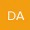What is the answer to this question? Which of these triangles obeys Pythagoras's theorem A. a triangle with side lengths 3,4,6 B. a triangle with side lengths 6,8,11 c. a triangle with side lengths 10,15,18 d. a triangle with side lengths 5,12,14

According to Pythagoras's theorem, in a right-angled triangle, the square of the length of the hypotenuse (the side opposite the right angle) is equal to the sum of the squares of the lengths of the other two sides.

Therefore, we need to check each triangle to see if it satisfies this condition.

A. 3^2 + 4^2 = 9 + 16 = 25, which is not equal to 6^2 = 36, so this is not a right-angled triangle.

B. 6^2 + 8^2 = 36 + 64 = 100, which is not equal to 11^2 = 121, so this is not a right-angled triangle.

C. 10^2 + 15^2 = 100 + 225 = 325, which is not equal to 18^2 = 324, so this is not a right-angled triangle.

D. 5^2 + 12^2 = 25 + 144 = 169, which is equal to 14^2 = 196, so this is a right-angled triangle.

Therefore, the answer is d. a triangle with side lengths 5,12,14.So is a triangle with side lengths 5,12,14 a right angled triangle?

Yes, a triangle with side lengths 5, 12, and 14 is a right-angled triangle because it satisfies Pythagoras's theorem.

5^2 + 12^2 = 25 + 144 = 169, which is equal to 14^2, the square of the length of the longest side. This means that the angle opposite the side of length 14 is a right angle, and hence the triangle is a right-angled triangle.